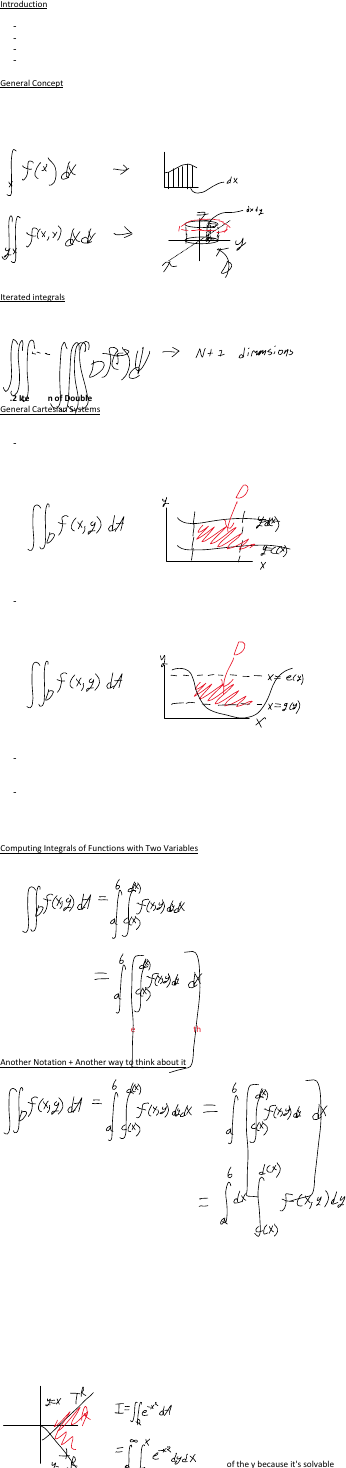Study Guides (380,000)
CA (150,000)
Western (10,000)
AMATH (40)
Final

Applied Mathematics 2277A/B Final: Final Studying Ch 14

Department
Applied Mathematics
Course Code
AMATH 2277A/B
Professor
C. Essex
Study Guide
Final

This preview shows page 1. to view the full 4 pages of the document.14.1 Double Integrals
Introduction
General coordinate spaces
-
General elements of length
-
General domain
-
General objects
-
"We need to define general properties of integrals to find repeated integrals"
In a function of 1 variable, we integrate it in 2D. If we have a function of two variables, in
order to integrate it we gotta expand it into 3 dimensions. The way that we do that is by
changing the differential of integration so we integrate over little 3D prisms instead of 2D
rectangles.
Iterated integrals
We can extend this concept into integrating N variables in N+1 dimensions
14.2 Iteration of Double Integrals in Cartesian Coordinates
General Cartesian Systems
There are types of domains within coordinate systems:
y-simple:
-
We say that the domain D in the xy-plane is y-simple if it is bounded by two vertical
lines
x-simple:
-
We say that the domain D in the xy-plane is x-simple if it is bounded by two
horizontal lines
If something is bound by a horizontal line and a vertical line, it is both x-simple and
y-simple
-
If something is neither x-simple or y-simple, it is called regular. These domains are
usually able to be broken down into subdomains that are either x-simple or y-
simple.
-
Computing Integrals of Functions with Two Variables
So we solving the integral inside the brackets, then the outside one
Another Notation + Another way to think about it
By moving the dx in front, we are demonstrating that this can be shown as being the
product of the integral of dy and the integral of dx. It's multiplication
14.3 Improper Integrals with double integration
Ordering matters.
Basically, you manipulate which integral you do first to make it so it can be solved
We've been doing this in
statics for years, but now
we're looking at the theory
behind it.
See? We're choosing to
solve the e for the x instead
of the y because it's solvable
Final Studying Ch 14
April 23, 2019
4:38 PM
Summaries Page 1